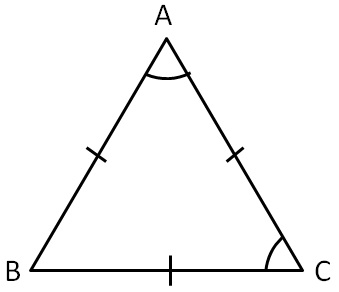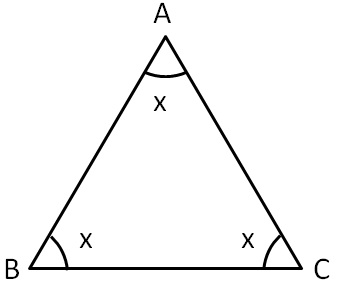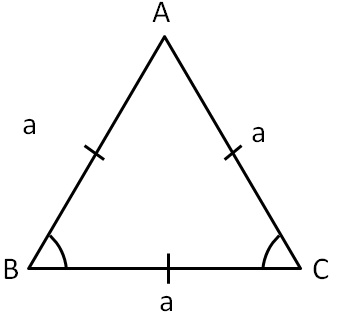Equilateral and Isoceles Triangle

Chapter 6 Class 7 Triangle and its Properties
Concept wise

In equilateral triangle,

• All sides are equal
• All angles all equal 60°

In equilateral ∆ ABC,

• AB = AC = BC
• ∠A = ∠B = ∠C = 60°### But, why are all angles 60°?

In equilateral triangle, all angles are equal.

Let ∠A = ∠B = ∠C = xIn ∆ABC

Sum of angles is 180°

∠A + ∠B + ∠C = 180°

x + x + x = 180°

3x = 180°

x = (180°)/3

x = 60°

So, ∠A = ∠B = ∠C = 60 °

Thus, all angles are 60°

### For equilateral trianglePerimeter = 3a

Check proof

Area = √3/4 a 2

Check proof

Learn in your speed, with individual attention - Teachoo Maths 1-on-1 Class